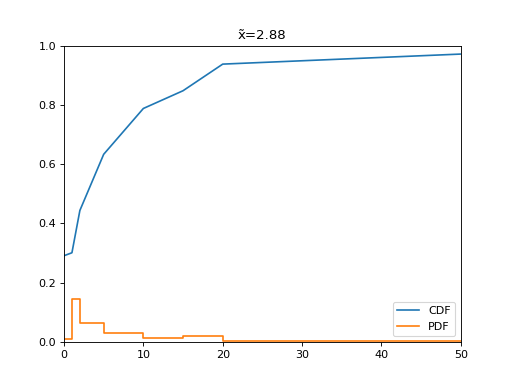# Distimate documentation¶

Distimate approximates and plots common statistical functions from histograms.

Distimate can aggregate empirical distributions of random variables. The distributions are represented as histograms with user-defined bucket edges. This is especially useful when working with large datasets that can be aggregated to histograms at database level.

```import distimate
import matplotlib.pyplot as plt

edges = [0, 1, 2, 5, 10, 15, 20, 50]
values = [291, 10, 143, 190, 155, 60, 90, 34, 27]
dist = distimate.Distribution.from_histogram(edges, values)

plt.title(f"x̃={dist.quantile(0.5):.2f}")
plt.xlim(0, 50)
plt.ylim(0, 1)
plt.plot(dist.cdf.x, dist.cdf.y, label="CDF")
plt.plot(dist.pdf.x, dist.pdf.y, label="PDF")
plt.legend(loc="lower right")
```Features:

• Histogram creation and merging
• Probability density function (PDF)
• Cumulative distribution function (CDF or ECDF)
• Quantile (percentile) function
• Pandas integration.

Distimate is hosted at GitHub and it can be installed from PyPI. This documentation is available online at Read the Docs.